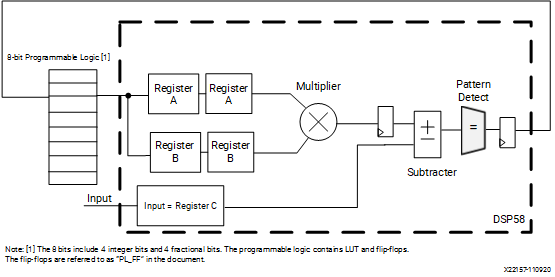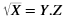# Square Root

## Versal ACAP DSP Engine Architecture Manual (AM004)

Document ID
AM004
Release Date
2022-09-11
Revision
1.2.1 English

The square root of an integer number can be calculated by successive multiplication and subtraction. It is similar to the subtraction method used to divide two numbers. The square root of an N-bit number will have N/2 (rounded-up) bits. If the square root is a fractional number, N/2 clocks are needed for the integer part of the result, and every following clock gives one bit of the fractional part. The logic needed to compute the square root is illustrated in the following figure. The calculation explained here is based on the assumption that there is one stage pipelining at the input of the multiplier.

Figure 1. Square Root LogicThe square root can be calculated as follows:Y is the integer part of the root and Z is the fraction part. Registers A and B refer to the registers found on the A and B inputs to DSP58 respectively and Register C refers to the registers found on the C input to DSP58. The steps to calculate are listed as follows.

1. Read the number into Register C. Set the register in external programmable logic (referred to as PL_FF) to `10000000`.
2. Calculate Register C – (PL_FF × PL_FF). C is a 16-bit value in the form `0000000C00000000`.
• If step 2 is positive, set PL_FF[(8-clock)] = 1, PL_FF[(8-clock) – 1] = 1
• If step 2 is negative, set PL_FF[(8-clock)] = 0, PL_FF[(8-clock) – 1] = 1
3. Repeat steps 2 and 3 until the required precision for the fractional part is reached.

In the case where there is only 1 stage pipelining to the input of the multiplier, four clock cycles are required to calculate the integer part of the value Y. The number of clock cycles required for the fraction part, Z, depends on the precision required. For an 8-bit value that has 4 bits for the integer part and 4 bits for the fractional part, the value in PL_FF after eight clock cycles includes the integer part given by the four MSBs and the fractional part given by the four LSBs. In the use case design, four additional pipeline stages are added for every 1-bit value to improve timing.

The reference design files associated with this use case are available in the square_root directory in the associated design archive file, am004-versal-dsp-engine.zip.